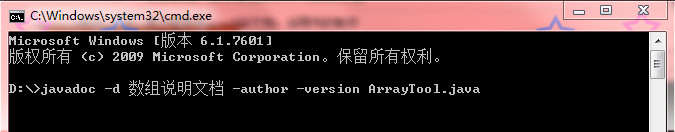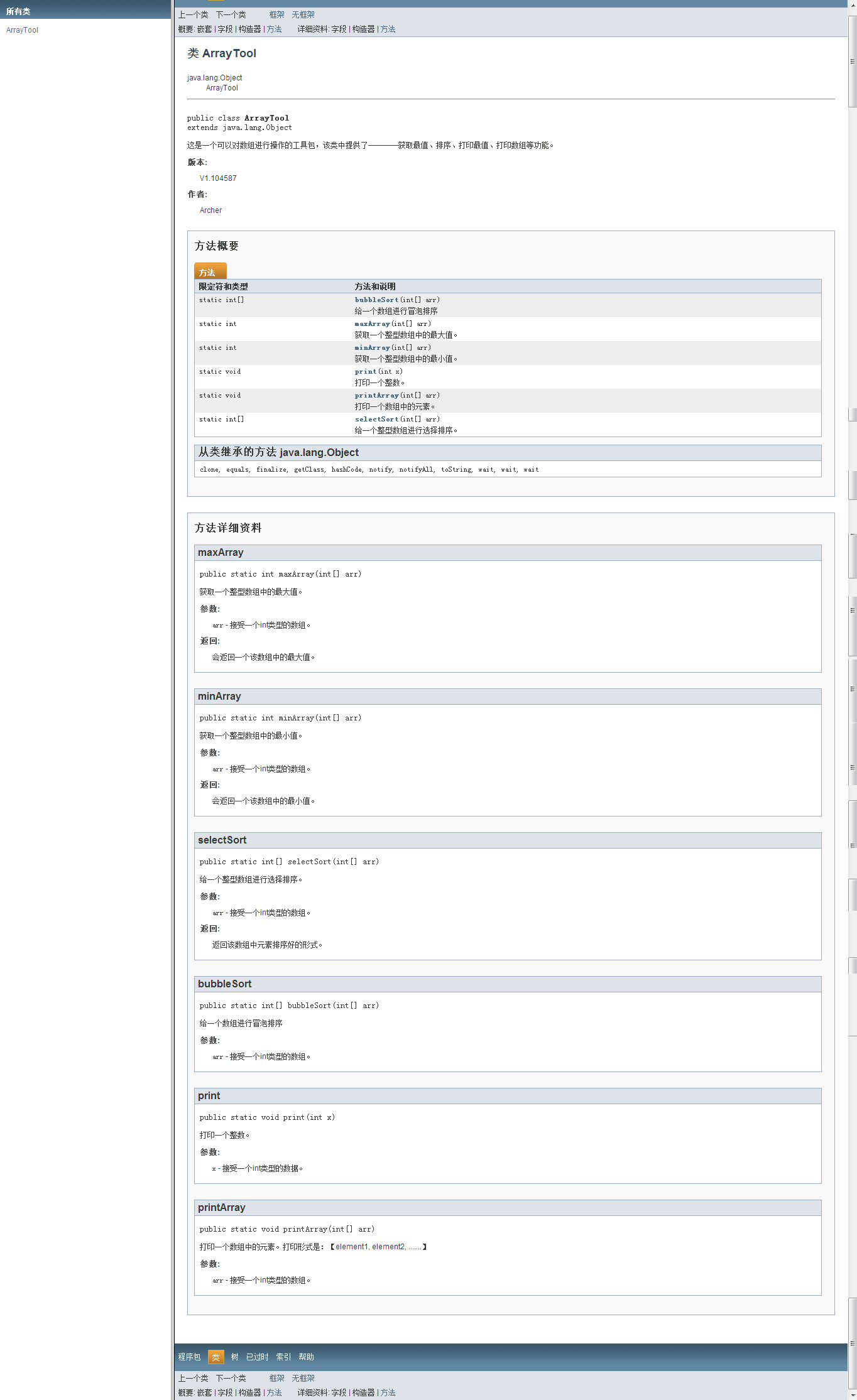# 一.API文档(Aplication Program Interface)

## 2.制作一个程序的说明文档

### 2.1 程序的代码部分书写格式如下

/**

@author Archer
@version V1.104587
*/
public class ArrayTool
{															//带有public的工具都被文档注释所标注，因为这样都能
int o = 100;											//提取出去
/**
空参数的构造函数。
*/
private ArrayTool(){}
/**
获取一个整型数组中的最大值。
@param arr 接受一个int类型的数组。
@return 会返回一个该数组中的最大值。
*/
public static int maxArray(int[] arr){
int max = 0;
for (int x = 0;x < arr.length-1;x++ )
{
if (arr[x] < arr[x+1])
{
max = arr[x+1];
}
}
return max;
}
/**
获取一个整型数组中的最小值。
@param arr 接受一个int类型的数组。
@return 会返回一个该数组中的最小值。
*/
public static int minArray(int[] arr){
int min = 0;
for (int x = 0;x <arr.length-1 ;x++ )
{
if (arr[x] < arr[x+1])
{
min = arr[x];
}
}
return min;
}
/**
给一个整型数组进行选择排序。
@param arr 接受一个int类型的数组。
@return 返回该数组中元素排序好的形式。
*/
public static int[] selectSort(int[] arr){
for (int x = 0;x < arr.length-1;x++)
{
for (int y = x+1;y <arr.length ;y++ )
{
if (arr[x] > arr[y])
{
swap(arr[x],arr[y]);
}
}
}
return arr;
}
/**
给一个数组进行冒泡排序
@param arr 接受一个int类型的数组。
*/
public static int[] bubbleSort(int[] arr){
for (int x = 0;x < arr.length-1 ;x++ )
{
for (int y = 0;y < arr.length-x-1 ;y++ )
{
if (arr[y] > arr[y+1])
{
swap(arr[y],arr[y+1]);
}

}
}
return arr;
}
/**
打印一个整数。
@param x 接受一个int类型的数据。
*/
public static void print(int x){
System.out.println(x);
}
/**
打印一个数组中的元素。打印形式是：【element1, element2, .......】
@param arr 接受一个int类型的数组。
*/
public static void printArray(int[] arr){
System.out.print("【");
for (int x = 0;x <arr.length ;x++ )
{
if (x < arr.length-1)
System.out.print(arr[x]+",");
else
System.out.print(arr[x]);

}
System.out.println("】");
}
/**
给两个变量进行数据的置换。
@param a 接受一个int类型的常量。
@param b 接受一个int类型的常量。
*/
private static void swap(int a,int b){
a = a ^ b;
b = b ^ a;
a = a ^ b;
}
}

### 2.2 运行时的汇编语言的书写### 2.3 说明书文档样式11-27
06-07
10-05428
11-03198
09-10178
10-17
02-23
03-06169
10-221778
04-13
10-17668
04-03309
04-17765
05-06450

### “相关推荐”对你有帮助么？

•非常没帮助
•没帮助
•一般
•有帮助
•非常有帮助被折叠的  条评论 为什么被折叠?到【灌水乐园】发言¥2 ¥4 ¥6 ¥10 ¥20余额支付 (余额：-- )扫码支付获取中扫码支付点击重新获取扫码支付1.余额是钱包充值的虚拟货币，按照1:1的比例进行支付金额的抵扣。
2.余额无法直接购买下载，可以购买VIP、C币套餐、付费专栏及课程。余额充值